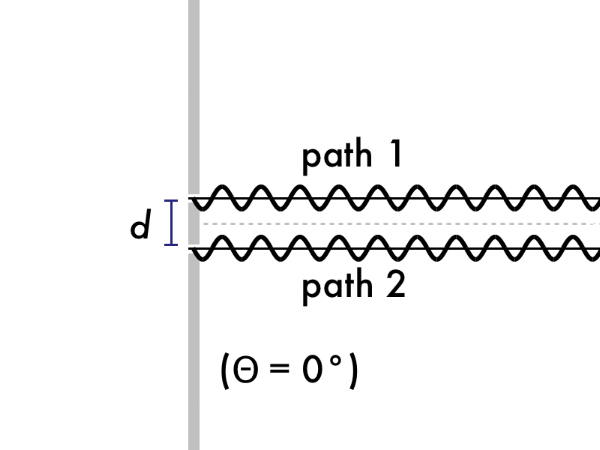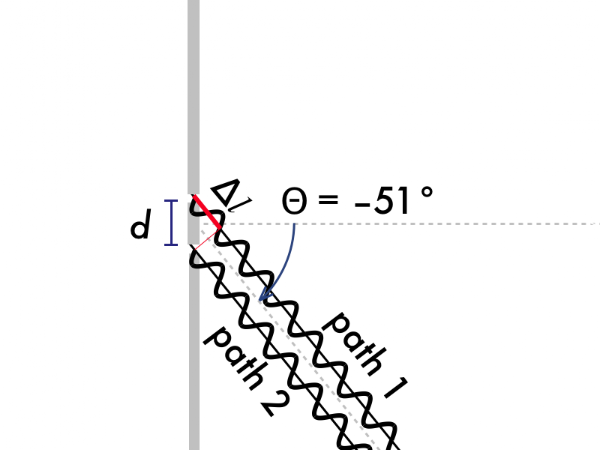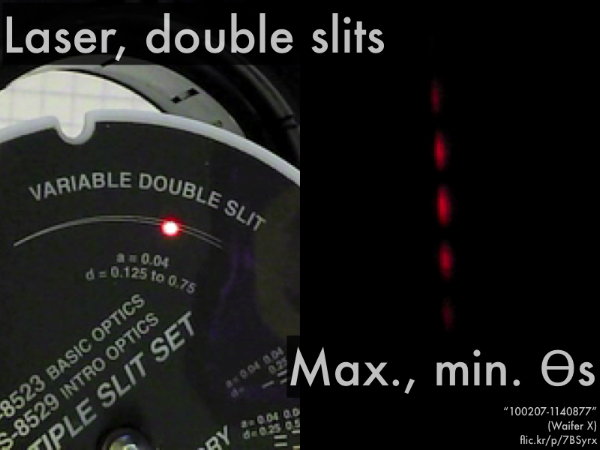## 20160223

### Presentation: double-slit interferenceHere we have microwaves (as discussed previously, a long wavelength form of electromagnetic radiation) from two side-by-side in-phase sources, interfering at a detector that can be moved at various locations to detect their interference, whether constructive or destructive (as translated into an audio signal). (Video link: "MIT Physics Demo--Microwave Interference.")In the previous presentation we discussed the conditions for constructive or destructive interference for waves (of the same wavelength) due to phase and/or path differences. In this presentation we discuss the very specific case of waves (again, of the same wavelength) from two side-by-side in-phase sources, which we will see has been classically called "double-slit" interference.First, path-length differences.The waves we are considering will come from two sources that are in phase, so we do not need to concern ourselves with the out of phase sources. Since source phase differences don't matter here--only path differences--then we must pay careful attention to the difference in path length: how much longer the wave from one source travels than the wave from the other source, as they reach and interfere at the position of the detector, as it moves from side-to-side.We are going to make the assumption that the detector is sufficiently (approaching infinitely?) far away from the two sources (spaced apart by a distance d) that the two waves will travel along a parallel path 1 and path 2. Then the location of the detector can be specified merely by the angle θ (where θ = 0° would be on the center line).

In this case, for the angle θ shown, waves travel longer along path 2. How much longer the waves travel along this longer path can be given by the relation ∆l = dsinθ. (There is a trigonometry derivation using the right triangle for this relation, but the focus here is on relating ∆l with the resulting constructive interference (maxima) or destructive interference (minima), and later on during problem-solving we'll use the ∆l = dsinθ relation to find these maxima and minima θ angles, without worrying too much about how to derive this ∆l = dsinθ relationship.)Here's the simple case (θ = 0°) where both waves leave their slits to travel equal distances to the distant detector towards the right. Do the waves start from their slits in phase or out-of-phase? Is the path difference a whole wavelength or a half-wavelength? Does constructive interference (maxima) or destructive interference (minima) occur at the detector?In this case, both waves leave their slits to travel unequal distances to the distant detector towards the right, located at an angle of θ = +23° off the (dashed) center line, such that path 1 is shorter than path 2. Do the waves start from their slits in phase or out-of-phase? Is the path difference a whole wavelength or a half-wavelength? Does constructive interference (maxima) or destructive interference (minima) occur at the detector? What would happen if the detector were instead placed at θ = –23° (same angle but on the other side of the center line)?Now in this case, both waves leave their slits again to travel unequal distances to the distant detector towards the right, located at an angle of θ = –51° off the (dashed) center line, such that path 1 is longer than path 2. Do the waves start from their slits in phase or out-of-phase? Is the path difference a whole wavelength or a half-wavelength? Does constructive interference (maxima) or destructive interference (minima) occur at the detector? What would happen if the detector were instead placed at θ = +51° (same angle, but on the other side of the center line)?Second, where we are going with this path-length difference relation: locating where these two sources interfere constructive (maxima) or destructively (minima).As discussed before, for in phase sources, the difference in path length will be some integer m multiple of a wavelength for constructive interference, or will be some integer and a half (m + 1/2) multiple of wavelength for destructive interference.So now let's put in our approximation for the path difference ∆l = dsinθ, for two waves from side-by-side sources reaching a (distant) detector located at an angle θ. What we will wind up with is a relation between the angle θ that a distant detector is located at, and the condition for either constructive (maxima) or destructive (minima) interference to occur. So given the wavelength λ of the two side-by-side sources, and the separation distance d between the two side-by-side sources, then plugging in different integer m values (0, ±1, ±2, ±3, etc.) allows us to solve for different θ angles where either constructive (maxima) or destructive (minima) interference occurs.You will demonstrate this for yourselves in recreating a classic experiment in laboratory. Using laser light (of a given wavelength λ) that illuminates two very closely spaced together slits (two in-phase sources spaced a distance d apart), there will appear bright (maxima, or constructive interference) regions and dark (minima, or destructive interference) regions on a screen (the detector) at certain θ angles, as predicted by the double-slit interference maxima/minima equations.